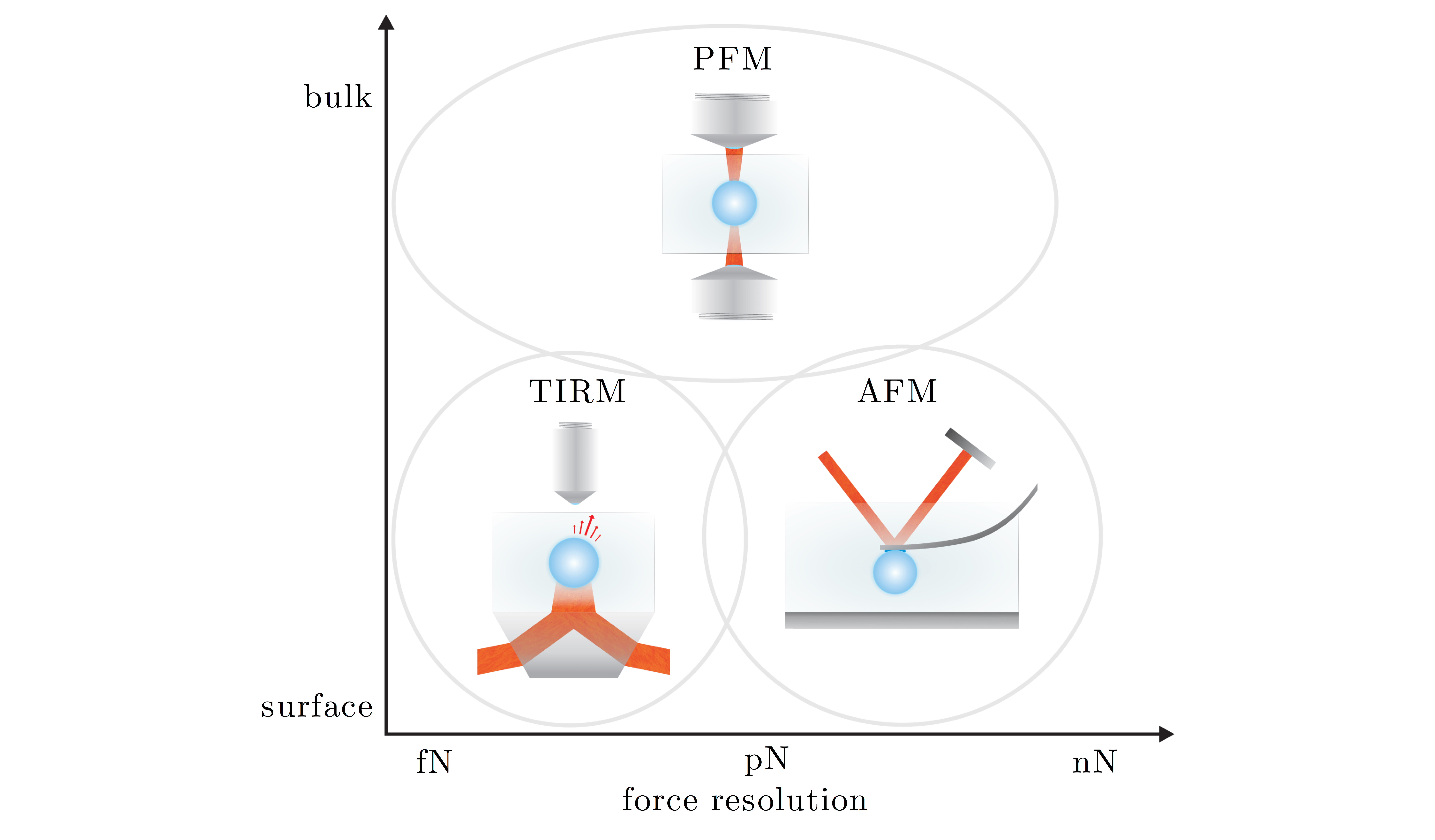# Chapter 10 — Photonic Force Microscope

One of the most successful applications of optical tweezers has been the measure- ment of small forces: since the optical restoring force is elastic, at least for most standard optical tweezers configurations, it is possible to measure the force acting on an optically trapped particle by multiplying its measured displacement from its equilibrium position and the optical trap stiffness. As shown in Fig. 10.1, the forces measurable by such a photonic force microscope (PFM) are typically in the range of tens of piconewtons down to few femtonewtons. Since these forces are comparable to most forces acting between biomolecules, the PFM has been extremely success- ful at measuring such forces. In this Chapter, we will review how the PFM can be employed to measure torques as well as forces, how the presence of surfaces or other particles affects the force measurement process and how much is the influence of non-conservative effects. Finally, we will describe how the optical force can be directly measured from the scattering associated with the trapping process.

10.1  Scanning probe techniques

10.2  Photonic torque microscope

10.3  Force measurement near surfaces
10.3.1  Equilibrium distribution method
10.3.2  Drift method

10.4  Relevance of non-conservative effects

10.5  Direct force measurement

Problems

References